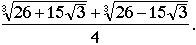Cardano's Method

Cardano's method provides a technique for solving the general cubic equation

ax3 + bx2 + cx + d = 0

in terms of radicals. As with the quadratic equation, it involves a "discriminant" whose sign determines the number (1, 2, or 3) of real solutions. However, its implementation requires substantially more technique than does the quadratic formula. For example, in the "irreducible case" of three real solutions, it calls for the evaluation of the cube roots of complex numbers.

In outline, Cardano's methods involves the following steps:

1. "Eliminate the square term" by the substitution y = x + b/3a. Rather than keeping track of such a substitution relative to the original cubic, the method often begins with an equation in the reduced form

x3 + px + q = 0.

2. Letting x = u+v, rewrite the above equation as

u3 + v3 +(u+v)(3uv + p) + q = 0.

3. Setting 3uv + p = 0, the above equation becomes u3 + v3 = -q. In this way, we obtain the system

u3 + v3 = -q

u3v3 = -p3/27.

Since this system specifies both the sum and product of u3 and v3, it enables us to determine a quadratic equation whose roots are u3 and v3. This equation is

t2 + qt -p3/27

with solutionsIn order to find u and v, we are now obligated to find the cube roots of these solutions. In the case

27q2 + 4p3 < 0

this entails finding the cube roots of complex numbers.

Even in the case 27q2 + 4p3 > 0, there are some unexpected wrinkles. These are illustrated by the equation

x3 + x2 - 2 = 0

for which x = 1 is clearly a solution. Although Cardano's method enables one to find this root without confronting cube roots of complex numbers, it displays the solution x = 1 in the rather obscure formIt is against this historical background that Chapter I of IADM develops an iteration-based alternative to Cardano's method at the pre-calculus level, one that is derived from "a Babylonian technique for finding cube roots."